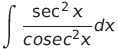Select Page

# CBSE Answers for MCQ 12 Science Maths Inverse Trigonometric Functions in English

CBSE Answers for MCQ 12 Science Maths Inverse Trigonometric Functions in English to enable students to get Answers in a narrative video format for the specific question.

Expert Teacher provides CBSE Answers for MCQ 12 Science Maths Inverse Trigonometric Functions through Video Answers in English language. This video solution will be useful for students to understand how to write an answer in exam in order to score more marks. This teacher uses a narrative style for a question from Inverse Trigonometric Functions not only to explain the proper method of answering question, but deriving right answer too.

Please find the question below and view the Answer in a narrative video format.

Question:

## Similar Questions from CBSE, 12th Science, Maths, Inverse Trigonometric Functions

Question 1 : Solve for(View Answer Video)

Question 2 : Find the greatest and least values of(View Answer Video)

Question 3 : Iffind x. (View Answer Video)

Question 4 : Write in the simplest form:(View Answer Video)

Question 5 : Ifthen find x. (View Answer Video)

### Integrals

Question 1 : Evaluate :(View Answer Video)

Question 2 : Find:Question 3 : Write the value of :(View Answer Video)

Question 4 : Write the value of:(View Answer Video)

Question 5 : Letfor every real number, x, where [x] is the greatest integer less than or equal to x.
Evaluate :(View Answer Video)

### Three Dimensional Geometry

Question 1 : Find the co-ordinates of the point where the line through the points A(3, 4, 1) and B(5, 1, 6) crosses the XY-plane. Also find angle which this line makes with the XZ-plane. (View Answer Video)

Question 2 : Find the co-ordinates of the point, where the lineintersects the plane x-y+z-5=0. Also find the angle between the line and the plane. (View Answer Video)

Question 3 : A plane makes intercepts -6, 3, 4 respectively on the co-ordinte axes. Find the length of the perpendicular from the origin on it. (View Answer Video)

Question 4 : Find the angle between the linesand(View Answer Video)

Question 5 : Find the vector and cartesian equations of the plane passing through the point (-1, 3, 2) and perpendicular to each of the planes x + 2y +3z = 5 and

### Vector Algebra

Question 1 : Find the angle between the vectorsand(View Answer Video)

Question 2 : If a line has direction ratios 2, -1, -2, then what are its direction cosines?   (View Answer Video)

Question 3 : Find the direction of the vector(View Answer Video)

Question 4 :  Find the scalar quantities from the following:
(i) 10 kg          (ii) 2 m north-west          (iii)(iv) 40 W         (v)(vi)(View Answer Video)

Question 5 : Find the scalar quantities from the following:
(i) Time period          (ii) Distance          (iii) Force